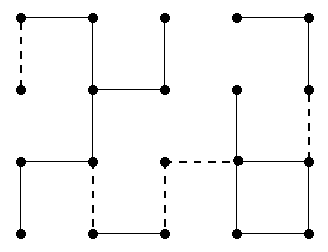Printed Circuit

PROBLEM

A printed circuit is a board that consists of nodes and wire segments connecting pairs of nodes. We consider a special kind of printed circuits where the nodes are arranged in a rectangular grid, and the wire segments connect (vertically or horizontally) adjacent nodes only. A printed circuit is called connected if any two distinct nodes are connected with a series of wire segments. Given is a printed circuit where wire segments already connect several adjacent nodes. We have to add new wire segments in order to make the printed circuit connected. The cost of a new wire segment is 1 if it is vertical and 2 if it is horizontal in the grid.Figure 1      Figure 2

You are to write a program that computes a least cost completion of a given circuit. Your program should solve the following three subtasks:

1. Determine the number of new wire segments of a least cost completion.
2. Compute the value of the least cost.
3. Produce a list of wire segments of a least cost completion.

INPUT

The first line of the file circuit.in contains two integers, N and M. N (1<=N<= 100) is the number of rows and M (1<= M<= 100) is the number of columns in the grid. The nodes of the circuit are identified by their coordinates; the node in the upper left corner is at (1,1), and the node in the lower right corner is at (N, M). Each of the next N lines contains M integers. The number in row i and column j of these lines describes the wire segments between the pair of nodes (i, j) and (i+1, j), and also between the pair of nodes (i, j) and (i, j+1) in the following way.

• 0 means that neither the pair of nodes (i, j) and (i+1, j) nor the pair of nodes (i, j) and (i, j+1) are connected by wire segments.
• 1 means that only the pair of nodes (i, j) and (i+1, j) is connected by a wire segment.
• 2 means that only the pair of nodes (i, j) and (i, j+1) is connected by a wire segment.
• 3 means that both the pair of nodes (i, j) and (i+1, j), and the pair of nodes (i, j) and (i, j+1) are connected by wire segments.

Note that not all 4 values are valid at certain positions (e.g. at position (N, M) only value 0 is valid).

OUTPUT

The first line of the file circuit.out should contain two integers, K and V. The integer K is the number of new wire segments of a least cost completion; and the integer V is the value of the least cost. The rest of the file must contain K lines; the list of wire segments of a least cost completion (in arbitrary order). Each line must contain three numbers describing exactly one new wire segment: i, j and d, where (i, j) are the coordinates of a node, and d is either 1 or 2. 1 means that the new wire segment connects the nodes (i, j) and (i+1, j), and 2 means that the new wire segment connects the nodes (i, j) and (i, j+1)

EXAMPLE INPUT AND OUTPUT

The example input file corresponds to the circuit in Figure 1. The example output file is a possible least cost completion, and is depicted in Figure 2.

 circuit.in circuit.out 4 5 2 1 1 2 1 0 3 0 1 0 3 0 0 3 1 0 2 0 2 0 5 6 1 1 1 3 2 1 3 3 1 3 3 2 2 5 1